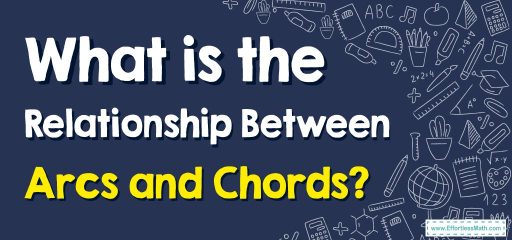# What is the Relationship Between Arcs and Chords?

Within the graceful confines of a circle, arcs and chords dance in tandem, establishing relationships and patterns that have intrigued mathematicians for centuries. While arcs define sections of a circle's boundary, chords are the linear segments that connect two points on that boundary. Their interplay defines various principles of circle geometry. This blog post provides a comprehensive exploration of the nuances of arcs and chords and their intricate interrelation.## Step-by-step Guide: Arcs and Chords

Definitions:

• Arc: An arc is a continuous segment of a circle’s circumference.
• Chord: A chord is a straight line segment whose endpoints lie on the circle. Note: The diameter is the longest chord of a circle.

Properties of Chords and Arcs:

• Chords that are equidistant from the center of a circle are equal in length.
• Equal chords of a circle subtend equal angles at the center.
• The perpendicular bisector of a chord passes through the circle’s center.

Relationship between Chords and Arcs:

• Equal chords intercept equal arcs.
• The angle subtended by an arc at the center is double the angle subtended by it at any point on the remaining part of the circle.

Calculating Length of Chords:
If we know the arc and radius, the chord’s length, $$c$$, can be approximated using:
$$c \approx 2r \sin\left(\frac{\text{arc angle in radians}}{2}\right)$$

### Examples

Example 1:
Two chords, $$AB$$ and $$CD$$, of a circle are of equal length. If the arc intercepted by chord $$AB$$ measures $$80^\circ$$, what is the measure of the arc intercepted by chord $$CD$$?
Solution: Given that equal chords intercept equal arcs, the arc intercepted by chord $$CD$$ will also measure $$80^\circ$$.

Example 2:
In a circle, an arc intercepts an angle of $$40^\circ$$ at the boundary. What is the angle subtended by this arc at the center?
Solution: The angle subtended by an arc at the center is double the angle subtended on the boundary. Therefore, the central angle is $$2 \times 40^\circ = 80^\circ$$.

Example 1:
Find the length of a chord that intercepts an arc of $$60^\circ$$ in a circle with a radius of $$10 \text{ cm}$$.
Solution: Convert the angle to radians: $$60^\circ \times \frac{\pi}{180} = \frac{\pi}{3} \text{ radians}$$. Using the formula:
$$c \approx 2 \times 10 \times \sin\left(\frac{\pi}{6}\right) \approx 10 \sqrt{3} \text{ cm}$$

### Practice Questions:

1. In a circle with a radius of $$7 \text{ cm}$$, what is the approximate length of a chord that intercepts an arc of $$90^\circ$$?
2. If an arc subtends an angle of $$30^\circ$$ at the boundary of a circle, what angle does it subtend at the center?

1. $$c \approx 2 \times 7 \times \sin(45^\circ) \approx 9.9 \text{ cm}$$
2. $$2 \times 30^\circ = 60^\circ$$

### What people say about "What is the Relationship Between Arcs and Chords? - Effortless Math: We Help Students Learn to LOVE Mathematics"?

No one replied yet.

X
30% OFF

Limited time only!

Save Over 30%

SAVE $5 It was$16.99 now it is \$11.99